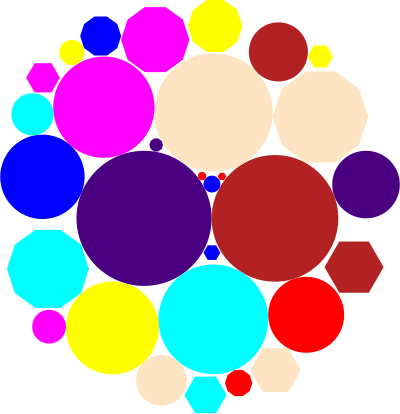circle-packing-0.1.0.6: Simple heuristic for packing discs of varying radii in a circle

Optimisation.CirclePacking

Contents

Description

Synopsis

# Documentation

packCircles :: (a -> Double) -> [a] -> [(a, (Double, Double))] Source #

packCircles takes a list of circles and a function that yields the radius of the circle.

It returns a list of all circles, in unspecified order, together with coordinates such that they do not overlap but sit as tight as possible, filling a large circle.

Finding the optimal solution to this is NP hard, so only heuristics are feasible. This particular implementation is neither very good nor very fast, compared to the state of the art in research. Nevertheless it is simple to use and gives visually acceptable results.

The heuristics begins by placing the largest circle first, and the next-to-largest next to it. From then on it adds circles by considering all points where the circle to be added would touch two circles but overlap with none, and picks the one that is closest to the center of mass of the current placements.

# Example

The following code demonstrates how one can use packCircles together with the diagrams library:

import Diagrams.Prelude
import Diagrams.Backend.SVG.CmdLine

import Optimisation.CirclePacking

colorize = zipWith fc $cycle [red,blue,yellow,magenta,cyan,bisque,firebrick,indigo] objects = colorize$
[ circle r  | r <- [0.1,0.2..1.6] ] ++
[ hexagon r | r <- [0.1,0.2..0.7] ] ++
[ decagon r | r <- [0.1,0.2..0.7] ]

-- Just a approximation, diagram objects do not have an exact radius
radiusApproximation o = maximum [ radius (e (CircleFrac alpha)) o | alpha <- [0,0.1..1.0]]

main = defaultMain $position$ map (\(o,(x,y)) -> (p2 (x,y),o)) \$
packCircles radiusApproximation objects

This generates the following SVG file (if your browser manages to display it):